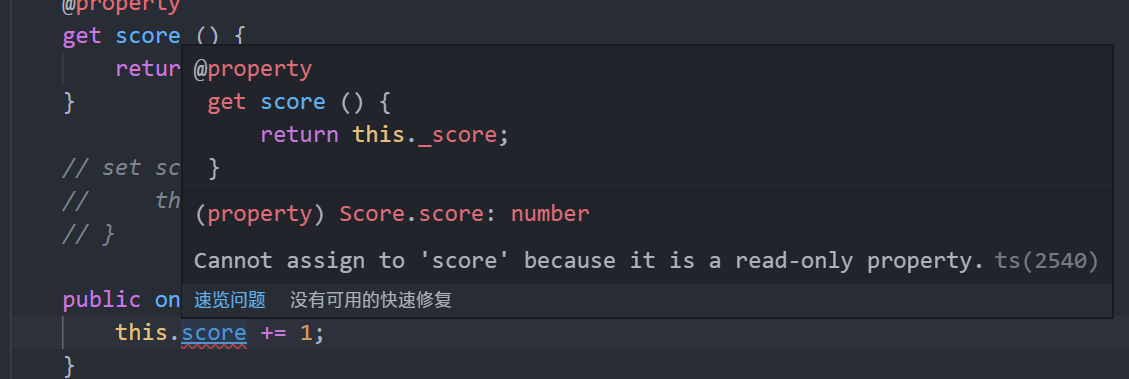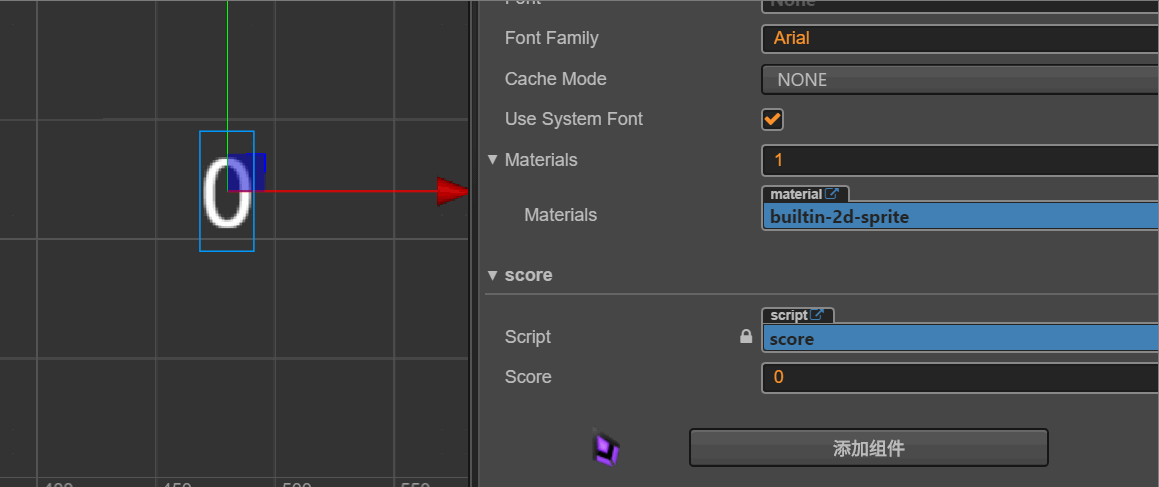# 置顶CocosCreator开发中为什么get/set如此重要？精选热门

### 正文

#### 案例

##### 案例一

``````public score: number = 0;
``````

##### 案例二

``````public score: number = 0;
``````

##### 案例三

``````this.score += 1;
this.getComponent(cc.Label).string = this.score + '';
``````

#### get/set的写法

JavaScript

``````cc.Class({
extends: cc.Component,

properties: {
score: {
get: function () {
return this._score;
},
set: function (value) {
this._score = value;
}
}
},

},

});
``````

TypeScript

``````const {ccclass, property} = cc._decorator;

@ccclass
export default class Score extends cc.Component {

private _score: number = 0;

@property
get score () {
return this._score;
}

set score (value) {
this._score = value;
}

}

}
``````

#### 只读方案

TypeScript

``````const {ccclass, property} = cc._decorator;

@ccclass
export default class Score extends cc.Component {

private _score: number = 0;

@property
get score () {
return this._score;
}

// set score (value) {
//     this._score = value;
// }

this.score += 1;
}

}
``````#### 限制修改

``````set score (value) {
// 最低为 0 分
if (value < 0) value = 0;
this._score = value;
}
``````#### MVVM实现

Model-View-ViewModel 数据与显示通过 ViewModel 联系起来。

TypeScript

``````set score (value) {
this._score = value;
this.getComponent(cc.Label).string = this._score + '';
}
``````TypeScript

``````const {ccclass, property} = cc._decorator;

@ccclass
export default class Score extends cc.Component {

private _score: number = 0;

@property
get score () {
return this._score;
}

set score (value) {
this._score = value;
this.getComponent(cc.Label).string = this._score + '';
}

// 编辑框输入事件
public inputScore (string: string ,editbox: cc.EditBox) {
let s = parseInt(string);
this.score = s;
}

}

``````• 灵活控制读写权限
• 为抽象做准备
• 数据可靠性
• 可以实现 MVVM

O(∩_∩)O~~

### 微信公众号